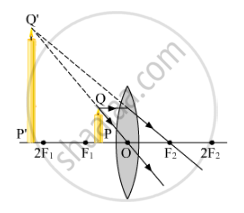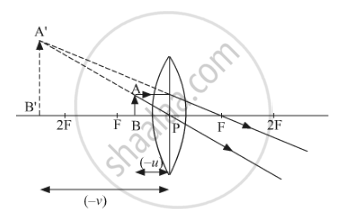# Draw a ray diagram to show the formation of an image by a convex lens when an object is placed in front of the lens between its optical centre and principal focus. - Science

(a) Draw a ray diagram to show the formation of an image by a convex lens when an object is placed in front of the lens between its optical centre and principal focus.

(b) In the above ray diagram, mark the object distance (u) and the image distance (v) with their proper signs (+ve or –ve as per the new Cartesian sign convention) and state how these distances are related to the focal length (f) of the convex lens in this case.

(c) Find the power of a convex lens which forms a real and inverted image of magnification –1 of an object placed at a distance of 20 cm from its optical centre.

#### Solution

(a)(b) The object distance (u) and the image distance (v) are indicated in the figure given below. Since both the image and the object lie in the direction opposite to the direction of the incoming ray, both of them will be negative. The relation between (u), (v) and (f) given by the lens formula is

1/f=1/v-1/u

As both (u) and (v) are negative, the above equation will be changed to

1/f=1/((-v))-1/((-u))

1/f=-1/v+1/u

1/f=1/u-1/v(c) Given:

u = −20 cm

m = −1

Since magnification is given as

m=v/u

rArrv=

Focal length can be calculated as

1/f=1/v=1/u=1/20-1/((-20))=1/10

rArrf=10

Thus, the power of the convex lens is

p=1/f(m)=100/10=10

Concept: Convex Lens
Is there an error in this question or solution?
2015-2016 (March) Delhi Set 1

Share